##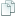Topic: Newb Question, Appropriate Terminology (Read 6032 times)previous topic - next topic

0 Members and 1 Guest are viewing this topic.
Newb Question, Appropriate Terminology
##### February 16, 2014, 04:09:04 pm
When creating statistics, I forgot what we call this:

Code: [Select]
` function Weapon (Name, Price, Atk)   {	this.Name = Name;	this.Price = Price;	this.Atk = Atk;} `

Is this a part of object oriented programming or prototypes, or do we just refer to it as "setting up for the arrays" that follow?

• Flying Jester
•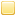• TurboSphere Developer
Re: Newb Question, Appropriate Terminology
##### Reply #1 – February 16, 2014, 04:29:37 pm
That's called a constructor, a function that constructs an objects as the `this`. It's kind of OOP, although you see constructors (of a sort) anywhere you have complex data structures, regardless of if they are used in an OO environment, or a procedural one, or anything else.Re: Newb Question, Appropriate Terminology
##### Reply #2 – February 16, 2014, 08:17:46 pm
What about when writing arrays, is there a name for the 0 inbetween the brackets?

Quote
blah.Atk

I know each array is giving a numerical value to identify which one they are, or to separate them from the others. Is there a name for the identifying number?

Apologies for these really amateur questions, haven't ever learned this stuff and kinda skipped over it until I started trying to write these tutorials.

• Flying Jester
•• TurboSphere Developer
Re: Newb Question, Appropriate Terminology
##### Reply #3 – February 16, 2014, 09:17:35 pm
That number is the index.

No problem, these aren't really questions that can be efficiently googled anyway.Re: Newb Question, Appropriate Terminology
##### Reply #4 – February 16, 2014, 11:34:11 pm
Say if I wanted to randomize the numbers inside an array, could I do it this way?

Code: [Select]
`  Team = new EnemysTeam(Math.random(0,3), Math.random(0,3), Math.random(0,3)); `

Will that randomize the elements each time the array is accessed, or only when the program starts up?

• Flying Jester
•• TurboSphere Developer
Re: Newb Question, Appropriate Terminology
##### Reply #5 – February 17, 2014, 12:01:39 am
The code you gave would change `team` to be an entirely new object. I kind of don't think that's what you want.

Also, that's not how Math.random() works. When in doubt, off to the MDN. It returns a floating point number between 0.0 and 1.0 (exclusive--it will never return exactly 1.0). You need to multiply by a factor and optionally add a constant, and/or use Math.floor() to convert to integer, to get a random number within a range that is not 0.0 <= n <1.0.

If you want to just get a randomized element of an array, something like this would do:
Code: [Select]
`function RandomizeArray(array){  return array[Math.floor(Math.random()*array.length)];}`

If you wanted an entire array that is a randomized version of another array, I would recommend making an array full of references to another array. That would be more work.Re: Newb Question, Appropriate Terminology
##### Reply #6 – February 17, 2014, 01:14:52 am
Had to concentrate a bit to follow that last bit, but I think I'm beginning to understand. This might get hard to follow, but just ask and I'll try my best to clear it up. Here's an overview:

So to make things simple, what I'm attempting to do is randomize each element inside the array.

However the randomization must be limited to only the specific numbers, which are indexes that identify certain specific enemies.
At the present moment, I'm simply substituting that problem to a limit in range between 0 and 3.

Quote
returns a floating point number between 0.0 and 1.0 (exclusive--it will never return exactly 1.0).

The problem here is that Math.random() in my other submission is returning decimal numbers. So the solution is:

Code: [Select]
` Math.floor( Math.random()*3 ); `

so that the randomization range will be limited to 3 and Math.floor will round the result to the nearest whole number?

However, if I just want to return a specific random element from an array I can just  use:

Code: [Select]
` function RandomizeArray(array){ return array[Math.floor( Math.random()*array.length )]; `

I have MDN pulled up currently, but I'm having a hard time understanding anything in their new layout which I'm starting to loathe.

IF you're multiplying array.length by Math.random(), is Math.random choosing between the elements in that array?
Or is Math.random simply being limited in range like if I were to simply multiply it by 3 like previously? <-- This one I think
Because array.length returns the number of elements in the array, it'll be similar to just multiplying 3 to Math.random.

Say this is the array:

Code: [Select]
`   Team = new EnemysTeam(3, 4, 1, 0); `

And I want to randomize each individual element in that array. I'm thinking I can start by making those global variables.

Code: [Select]
`   Team = new EnemysTeam(E1, E2, E3, E4); `

So I'll need to create a function which returns a result defining each individual variable.

I can take another separate array, and use it as a bank for Enemy Indexes.
Say this is the result:

Code: [Select]
` blah = new Indexes( 4, 3, 1. 0); `

Now how do I make a function return only one random element out of that array? I'm totally lost there.

Thinking about it, I'll have to use array.length and Math.random to select the element through place value, but how do I return that element?
Looking through MDN, can I use Array.find() in sphere? If so, I can probably do this:

Code: [Select]
` function FindElement() { var Result = Math.floor( Math.random() * blah.length )  return Result * blah.find } `

So that the result of Math.random returns the place value of the element that array.find will return.
Or does it not work like that? How do you even establish the place value for array.find to look for, if not through multiplication?

••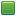• Senior Staff
• Wise Warrior
Re: Newb Question, Appropriate Terminology
##### Reply #7 – February 17, 2014, 04:35:44 am
Math.floor floors a number, so it always rounds down. 1.1 is 1, 1.9 is 1, and 1.0001 is also 1.

I see what you are trying to do and Jest isn't doing it. You want to randomize an array, not just get a random element. The easiest approach is to use what's called the knuth shuffle (see: Fisher-Yates algorithm). Here is an implementation.
Code: (javascript) [Select]
`function RandomizeArray(array) {    for (var i = array.length - 1; i > 0; i--) {        var j = Math.floor(Math.random() * i);        var temp = array[j];        array[j] = array[i];        array[i] = temp;    }}`

It works by randomizing elements that are not yet placed. Index i indicates which have been 'locked down' while index j is a random index of what's left. It randomly puts what's left into a locked in place. Then decrements the locked in place by one, and does it again until everything is random. Notice this algorithm performs very fast and efficient on known-length arrays.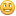Not to confuse you, but Math.floor(Math.random() * array.length) will return the correct random index. That's because an array is 0-based, and array.length starts it's count at 1. That means an arrays length is always 1 greater than the number of elements in an array. Realize the 'count' of items is different than an items index. So Math.random() always is less than 1 and is multiplied with an value 1 greater than the index. By flooring this value, we will never breach the edge of the array.

To show in detail:

[0, 1] -> indices 0, 1 but count of '2'.
Math.random() * 2 -> is between 0 and 1.999999999
Math.floor(Math.random() * 2) is between 0 and 1, the two indices.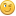If you use code to help you code you can use less code to code. Also, I have approximate knowledge of many things.

Sphere-sfml here
Sphere Studio editor here

Re: Newb Question, Appropriate Terminology
##### Reply #8 – February 17, 2014, 12:37:12 pm
So say I plug in the array:  Team = new EnemysTeam(3, 4, 1, 0);
Do I do it like this?

Code: [Select]
` function RandomizeArray(Team) { `

And in the For-Loop, here:

Code: [Select]
` for (var i = array.length - 1; i > 0; i--) { `

The High Cap: array.length - 1;
The Low Cap:  i > 0;

What it's doing is getting the length of the array, which is 4, then subtracting 1 until it becomes 0. Correct? (Result is always between 0 and 3)
But if we floor the next equation, doesn't that mean the range is only 0 to 2? Being that the largest integer from the For-Loop could only be 3.

Here's the part that confuses me a little:
So the Knuth Shuffle creates a new array with new randomized elements, and Index i is the results?
And Index j is what is left to be randomized for the function, or what numbers are left that weren't chosen?

Also wouldn't I need to array.splice to add the new results to array
because wouldn't leaving it like it is just keep replacing i with new results, instead of binding them to the array?

And if I want to return the array, do I just:  return array

So basically the function returns an array with randomized elements between 0 and 3.
Say if I want to use those elements as place value to find the elements in separate array.
Could I do that using array.find?

••• Senior Staff
• Wise Warrior
Re: Newb Question, Appropriate Terminology
##### Reply #9 – February 17, 2014, 01:12:31 pm
I'm sorry, but you are very confused!

Umm, to use the randomize array call it's this:
Code: (javascript) [Select]
`RandomizeArray(your_array);`

Math.floor is correct to use, Math.round should not be used for it. But I think I see a vbug in my code. The specification is 0 <= j <= i, and I don't have that. Mine is 0 <= j < i, so, to correct that:

Code: (javascript) [Select]
`        var j = Math.floor(Math.random() * (i + 1));`

We don't use Math.round since it may round up or down depending on the random number and we may get inconsistent results. Flooring it gives us 0 as a bottom-most value, whereas Math.round may not always do that.

Also, you don't need to return the array since it modifies the existing array. Arrays are like objects, they are passed by reference. That means when they changed in a function the changes are kept afterwards. They are not copied like how numbers are, for example. Knuth Shuffle does not create a new array, where does it do that?

Also, you don't need Array.splice, you can get and set values in an array with the indexer, like so:
Code: (javascript) [Select]
`var my_array = [];my_array = 1;my_array = 2;var m = my_array + my_array; // m = 3`

In the Knuth Shuffle, this part:
Code: (javascript) [Select]
`        var temp = array[j];        array[j] = array[i];        array[i] = temp;`

Just swaps two array elements. It temporarily puts what is at array[j] into temp, then rewrites array[j] with array [ i ], then you have to put temp back into array[ i ] since the original place had been rewritten. It's a very standard way of swapping two array values.If you did this:
Code: (javascript) [Select]
`var my_array = [];my_array = 1;my_array = 2;my_array = my_array;my_array = my_array;`

Then my_array is filled with 2, 2, and not 2, 1.Therefore the temp var is needed.
If you use code to help you code you can use less code to code. Also, I have approximate knowledge of many things.

Sphere-sfml here
Sphere Studio editor here

Re: Newb Question, Appropriate Terminology
##### Reply #10 – February 17, 2014, 02:04:11 pm
Well some things are cleared up.

 Error: I thought the Knuth Shuffle creates a new array, because I mistakenly thought all functions create new independent results.
Correction: However now I know existing arrays can be modified with functions that call them.

 Also understand why we shouldn't use Math.round, because it has too much variability to switch between two whole numbers.
We want hard concrete results that we will know, so we use Math.floor

Code: [Select]
`var my_array = [];     my_array = 1;my_array = 2;     var m = my_array + my_array; // m = 3 `

Isn't this combining the elements to get the result: var m = 3?

And in the Knuth Shuffle:
Code: [Select]
`var temp = array[j];array[j] = array[i];array[i] = temp; `

Isn't it swapping and overwriting the elements?
Instead of splicing more elements into one array?

For example, if j = 3 and i was once i = 0
Then now array is now array?

I was thinking more how do I get the array:

Code: [Select]
` var blah = ; `

to become:

Code: [Select]
` var blah = [0, 3]; `

Or is that what the Knuth Shuffle is doing? And I'm just really confused.

••• Senior Staff
• Wise Warrior
Re: Newb Question, Appropriate Terminology
##### Reply #11 – February 17, 2014, 04:10:27 pm
Oh, wait you want to add to an existing array? I thought you just wanted to randomize an existing array. Jest gave you an idea but it would invole creating new arrays, etc. The Knuth shuffle does it in 'one pass' so to speak.

This is what you want:
Code: (javascript) [Select]
`var enemies = [enemy1, enemy2, enemy3];// randomize the list:RandomizeArray(enemies);`

There's no need for anything else.Code: [Select]
`var my_array = [];     my_array = 1;my_array = 2;     var m = my_array + my_array; // m = 3 `

Isn't this combining the elements to get the result: var m = 3?

By combining you mean add, then yes. It finds the value at element 0 and adds it to the value at element 1. Since those values are 1 and 2 respectfully, they add up to 3. You can store other things as well in arrays such as enemy objects, strings, boolean values, etc.

And in the Knuth Shuffle:
Code: [Select]
`var temp = array[j];array[j] = array[i];array[i] = temp; `

Isn't it swapping and overwriting the elements?
Instead of splicing more elements into one array?

That part swaps an element. It doesn't splice so it doesn't add or remove from the array. It merely takes two items and swaps them. It's like looking at a list of coins, taking two random coins and swapping them. Do it with your hands in real life and you get the idea. It's just that in code you need a temporary value to hold onto the first item since the second overwrites it, and you no longer have access to it, so it's important to keep that around elsewhere.
If you use code to help you code you can use less code to code. Also, I have approximate knowledge of many things.

Sphere-sfml here
Sphere Studio editor here

Re: Newb Question, Appropriate Terminology
##### Reply #12 – February 17, 2014, 05:43:25 pm
Alright, I'm understanding this now. I is so happy.
However I need to explain what I'm trying to accomplish, but better terms.
I may get something wrong, so ask if something's not clear.

I have some idea on how I might be able to do it,
but I need to figure out what some things do before I lose the idea.

First, what does array.find do?
Can it select a specific element out of an array?
If so, how do I give it the place value of that element?

The True Problem:
[PART ONE:]

Code: [Select]
` var enemies = [enemy1, enemy2, enemy3, enemy4]; // <--The Bank for Enemy Indexes `

Each enemy# in the array represents an enemy's index.

There is only FOUR place values in the array. I need to randomly select ONE place value.
I must then use that random place value, to identify and return a specific number from that array.

That specific number can only be a number that is in that array.
Say I have this array:

Code: [Select]
` var enemies = [532, 49, 0, 86]; `

I can only return one of those specific numbers.
So I randomly choose a place value, then use that place value to find and return a number.

In other words, use Math.random to choose a specific number in that array. (through place value)
Then return that specific number.

[PART TWO:]
Once I have that very specific number, say it's 532 from the array above.
Then I must insert it into a new array, here:

Code: [Select]
` Team = new EnemysTeam( 532, E2, E3, E4 ); // <-- Enemy Indexes `

This is done by making E1 a global variable. var E1 = 0;
Then once I have my specific number, 532, I update the variable to become: var E1 = 532;

So I understand that by using the Knuth Shuffle, we can swap elements out for a brand new randomized element.
However I'm wanting to not replace the elements, but find and choose them. Randomly.
This is so I can use the element that's chosen, to be inserted in this array:

Code: [Select]
` Team = new EnemysTeam( E1, E2, E3, E4 ); // <-- Enemy Indexes `

Through global variables.

[SOLUTION:]
Instead we just use Math.random to find a number between 0 and 4. (That's our place value) Or using array.length+1;
Then we use that Place Value to find and choose a number out of the first array.
Once chosen, that number is returned and becomes Variable E1.
Thus, the E1 spot in the second array is now defined.

Then we have to put all this through a For-Loop, until all four spots have been defined. For(var i = 0, i >= 4, i++);
And we have finished our new list of random enemies.

. . . .

[NOTE:] This is a little hard for even me to follow, so please ask if something isn't making sense.
I'm so far out of my league that it doesn't surprise me that most of this might come off as crazy or illegible.

Re: Newb Question, Appropriate Terminology
##### Reply #13 – February 17, 2014, 08:07:36 pm
Alright, never mind.
I tested the Knuth Shuffle in Sphere, and it fixes the first part of my problem.
I was just really confused about the Knuth Shuffle, and still kinda am.

Here's the code:
Code: [Select]
`var enemies = [23, 45, 72, 84];function RandomizeArray(array) { for (var i = array.length - 1; i > 0; i--)  {  var j = Math.floor(Math.random() * (i + 1));  var temp = array[j];	  array[j] = array[i];  array[i] = temp; } return array[i]} `

This returns those specific elements in the array as I was wanting.

Problem: However, for some reason it throws me an "undefined" every now and then, and I'm not sure why.
Edit: Nvm, math error.

Re: Newb Question, Appropriate Terminology
##### Reply #14 – February 17, 2014, 08:41:44 pm
[PART TWO:]

Code: [Select]
`var E1 = 0;var E2 = 0;var E3 = 0; var E4 = 0;function RandomizeEnemies() {for (var b = 0; b i <= 4; b++) {  RandomizeArray(enemies) }} `

So I have the global variables and the For-Loop setup, how do I copy the results from each RandomizeArray() to each E# variable.
In my mind, I was thinking something like this:

Code: [Select]
`function RandomizeEnemies() {for (var b = 0; b i <= 4; b++) {  var "E" + b = RandomizeArray(enemies); }} `

But I'm positive that's not going to work, but not sure what else will.